# 12-240/Proofs in Vector Spaces

(diff) ← Older revision | Latest revision (diff) | Newer revision → (diff)

This page is intended for sharing/clarifying proofs. Here, you might add a proof, correct a proof, or request more detailed explanation of some specific parts of given proofs. To request an explanation for a proof, you may put a sign at that specific part by editing this page. For example:

...generating set as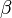$\beta$, so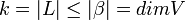$k = |L|\leq |\beta| = dimV$ ***(explanation needed, why? [or your question])*** since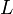$L$ is a some linearly independent...

## Theorems & Proofs

Theorem: Let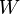$W$ be a subspace of a finite dimensional vector space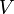$V$. Then$W$ is finite dimensional and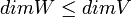$dimW \leq dimV$

Proof: Let$\beta$ be a basis for$V$. Then we know that$\beta$ is a finite set since$V$ is a finite dimensional. Then, for given a subspace$W$, let us construct a linearly independent set$L$ by adding vectors from$W$ such that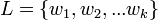$L=\{w_1,w_2, ... w_k\}$ is maximally linearly independent. In other words, adding any other vector from$W$ would make$L$ linearly dependent. Here, L has to be a finite set by the Replacement Theorem, if we choose the generating set as$\beta$, so$k = |L|\leq |\beta| = dimV$ since$L$ is a some linearly independent subset of$V$. Now we want to show that$L$ is a basis for$W$. Since$L$ is linearly independent, it suffices to show that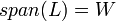$span(L)=W$. Suppose not: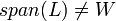$span(L)\neq W$. (We know that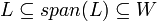$L \subseteq span(L) \subseteq W$ since$L$ is made of vectors from$W$.) Then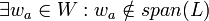$\exists w_a \in W : w_a \notin span(L)$ But this means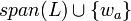$span(L)\cup \{w_a\}$ is linearly independent, which contradicts with maximally linearly independence of$L$. Therefore$span(L)=W$ and hence,$L$ is a basis for$W$

Replacement Theorem: Let$V$ be a vector space generated by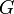$G$ (perhaps linearly dependent) where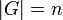$|G|=n$ and let$L$ be a linearly independent subset of$V$ such that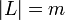$|L|=m$. Then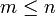$m \leq n$ and there exists a subset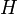$H$ of$G$ with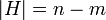$|H| = n-m$ and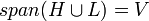$span(H \cup L)=V$.

Proof: We will prove by induction hypothesis on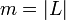$m=|L|$:

For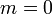$m = 0$: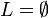$L = \emptyset$,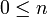$0 \leq n$ and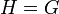$H=G$ so,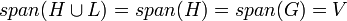$span(H \cup L) = span(H) = span(G) = V$

Now, suppose true for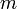$m$: